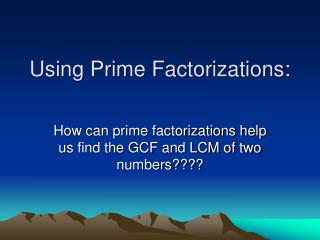DownloadDownload PresentationUsing Prime Factorizations:

# Using Prime Factorizations:

Download Presentation## Using Prime Factorizations:

- - - - - - - - - - - - - - - - - - - - - - - - - - - E N D - - - - - - - - - - - - - - - - - - - - - - - - - - -
##### Presentation Transcript

1. Using Prime Factorizations: How can prime factorizations help us find the GCF and LCM of two numbers????

2. Prime Factorization Defn: • The prime factorization of a number is a string of factors made up of only prime numbers.

3. The GCF: • Find the Prime Factorization of 24 and 60. • 24 = and 60 = • Work with your partner: • What would be the GCF of 24 and 60?

4. The GCF would be the product of the longest string of prime factors that the numbers have in common. For example, the longest string of factors 24 and 60 have in common is 2 x 2 x 3. So, the GCF of 24 and 60 is : 2 x 2 x 3 = 12

5. Now you try…. • Using the idea of prime factorization, find the GCF for: • 48 and 72 • 30 and 54

6. The LCM: • 24 = 2 x 2 x 2 x 3 and 60 = 2 x 2 x 3 x 5 • Work with your partner to come up with a way to find the LCM of 24 and 60 using their prime factorizations.

7. The least common multiple of two numbers is the product of the shortest string that contains the prime factorizations of BOTH numbers. • For example: the shortest string that contains the prime factorizations of 24 and 60 is: 2 x 2 x 2 x 3 x 5

8. Now you try…. • Find the least common multiple for: • 48 and 72 • 30 and 54

9. Follow-up: • The GCF of 25 and 12 is 1. Find two other pairs of numbers with a GCF of 1. Such pairs of numbers are said to be relatively prime.

10. The LCM of 6 and 5 is 30. Find two other pairs of numbers for which the LCM is the product of the numbers.

11. Find two pairs of numbers for which the LCM is small than the product of the two numbers. For example, the product of 6 and 8 is 48; the LCM is 24.

12. How can you tell from the prime factorization whether the LCM of two numbers is the product of the two numbers or is less than the product of the two numbers? EXPLAIN